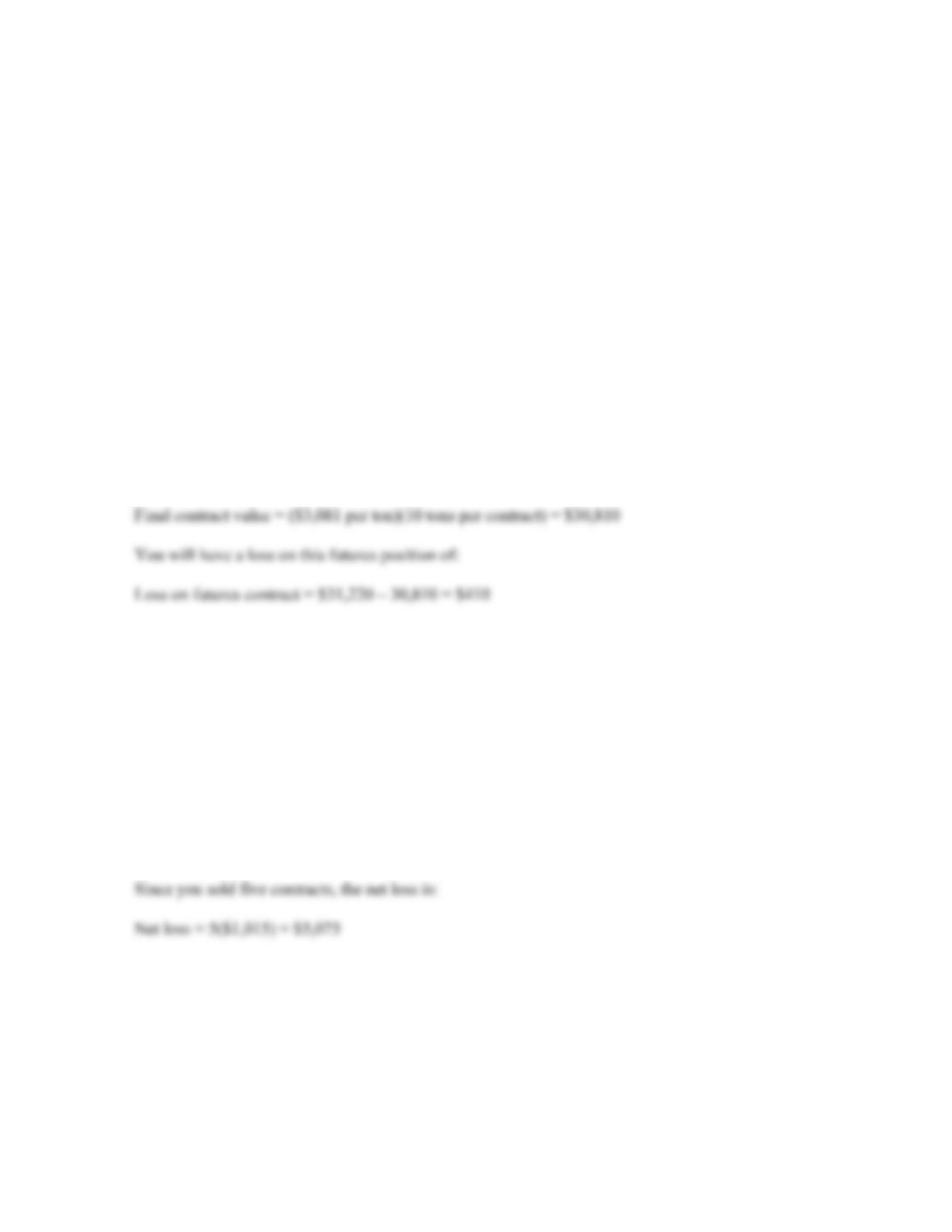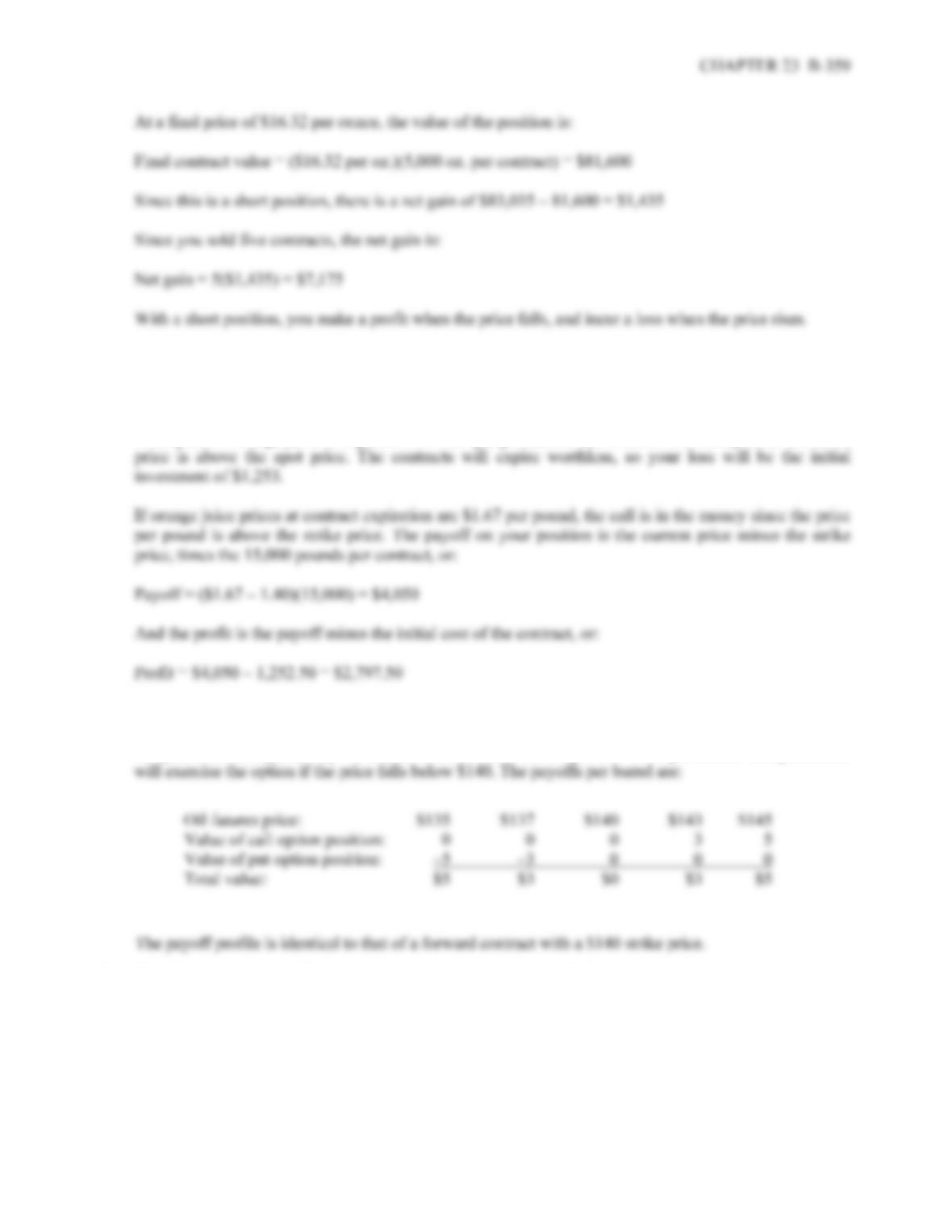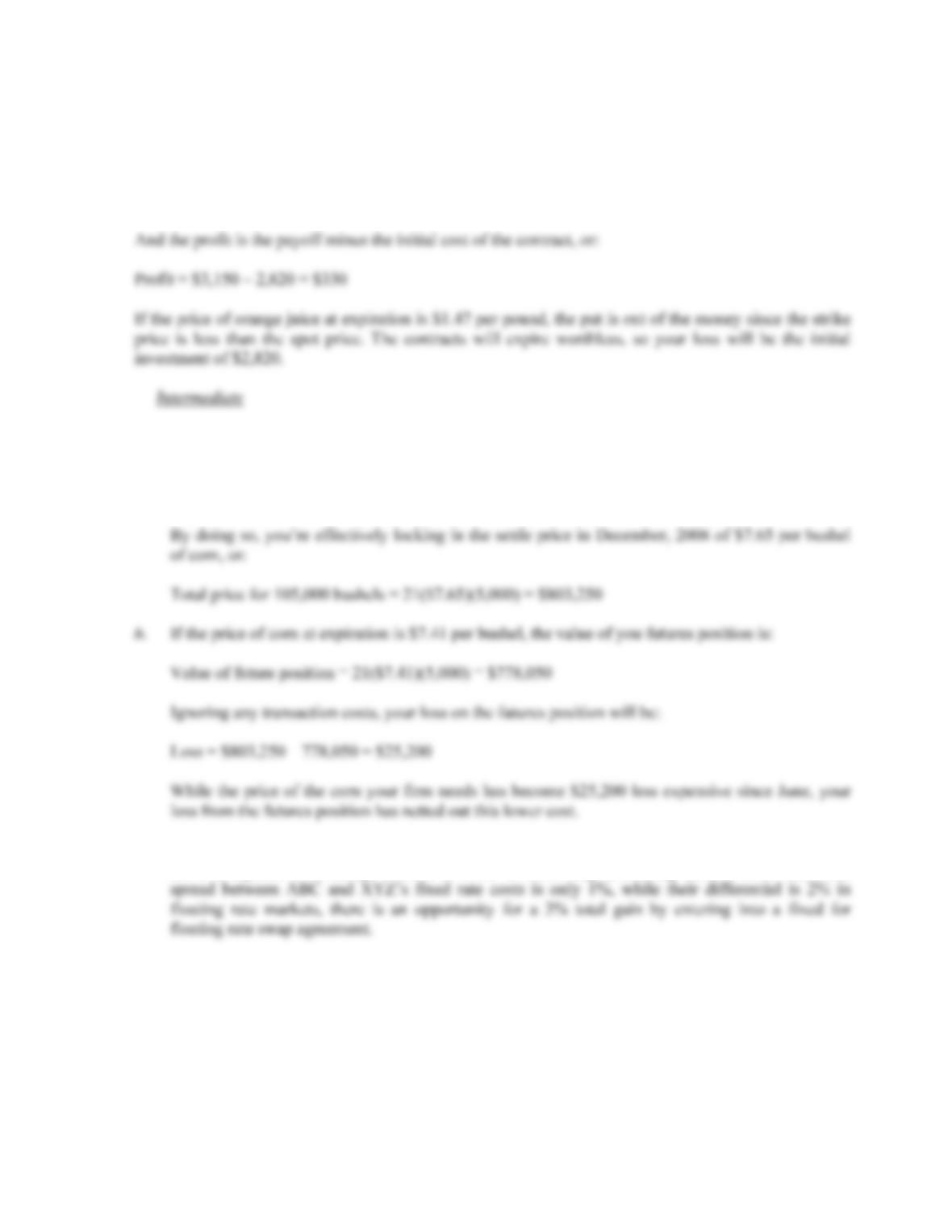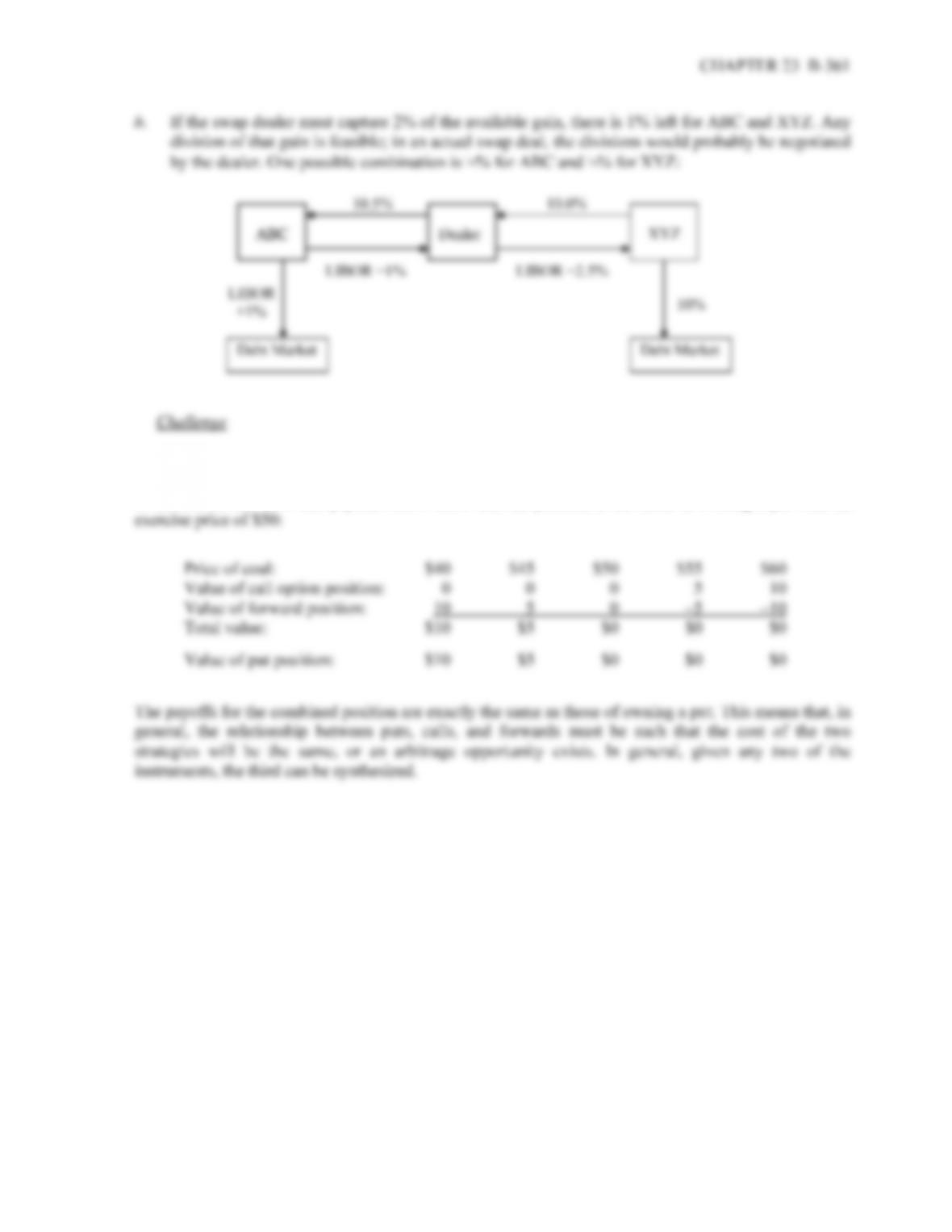Type
Quiz
Book Title
Fundamentals of Corporate Finance Standard Edition 9th Edition
ISBN 13
978-0073382395

### 978-0073382395 Chapter 23 Questions and Problems

April 3, 2019B-358 SOLUTIONS
14. Sysco must have felt that the combination of fixed plus swap would result in an overall better rate. In
other words, variable rate available via a swap may have been more attractive than the rate available
from issuing a floating-rate bond.
Solutions to Questions and Problems
NOTE: All end of chapter problems were solved using a spreadsheet. Many problems require multiple steps.
Due to space and readability constraints, when these intermediate steps are included in this solutions
manual, rounding may appear to have occurred. However, the final answer for each problem is found
without rounding during any step in the problem.
Basic
1. The initial price is \$3,122 per metric ton and each contract is for 10 metric tons, so the initial contract
value is:
Initial contract value = (\$3,122 per ton)(10 tons per contract) = \$31,220
And the final contract value is:
2. The price quote is \$16.607 per ounce and each contract is for 5,000 ounces, so the initial contract value
is:
Initial contract value = (\$16.607 per oz.)(5,000 oz. per contract) = \$83,035
At a final price of \$16.81 per ounce, the value of the position is:
Final contract value = (\$16.81 per oz.)(5,000 oz. per contract) = \$84,050
Since this is a short position, there is a net loss of:
\$84,050 – 83,035 = \$1,015 per contract3. The price quote is \$0.0835 per pound and each contract is for 15,000 pounds, so the cost per contract is:
Cost = (\$0.0835 per pound)(15,000 pounds per contract) = \$1,252.50
If the price of orange juice at expiration is \$1.29 per pound, the call is out of the money since the strike
4. The call options give the manager the right to purchase oil futures contracts at a futures price of \$140
per barrel. The manager will exercise the option if the price rises above \$140. Selling put options
obligates the manager to buy oil futures contracts at a futures price of \$140 per barrel. The put holder
5. The price quote is \$0.1880 per pound and each contract is for 15,000 pounds, so the cost per contract is:
Cost = (\$0.1880 per pound)(15,000 pounds per contract) = \$2,820.00B-360 SOLUTIONS
If the price of orange juice at expiration is \$1.14 per pound, the put is in the money since the strike price
is greater than the spot price. The payoff on your position is the strike price minus the current price,
times the 15,000 pounds per contract, or:
Payoff = (\$1.35 – 1.14)(15,000) = \$3,150
6. a. You’re concerned about a rise in corn prices, so you would buy December contracts. Since each
contract is for 5,000 bushels, the number of contracts you would need to buy is:
Number of contracts to buy = 105,000/5,000 = 21
7. a. XYZ has a comparative advantage relative to ABC in borrowing at fixed interest rates, while ABC
has a comparative advantage relative to XYZ in borrowing at floating interest rates. Since the8. The financial engineer can replicate the payoffs of owning a put option by selling a forward contract and
buying a call. For example, suppose the forward contract has a settle price of \$50 and the exercise price
of the call is also \$50. The payoffs below show that the position is the same as owning a put with an